Get instant live expert help with Excel or Google Sheets“My Excelchat expert helped me in less than 20 minutes, saving me what would have been 5 hours of work!”

#### Post your problem and you’ll get Expert help in seconds.

Your message must be at least 40 characters
Our professional Expert are available now. Your privacy is guaranteed.

# Excel Interview Questions and Answers – Excelchat

Many jobs require us to be experts in Excel. Entrepreneurs, freelancers, small and large businesses all use Microsoft Applications such as Word, Excel, and PowerPoint. We may be asked a few questions about Excel at that upcoming job interview. Therefore, we have put together some interview questions and answers. These outlined interview questions are to help us learn specific topics before seeking other areas of Excel.

## Microsoft Excel Interview Questions on Formats

### What are the most important data formats in Excel?

• Numbers: This includes the decimals, fractions, separators and many more. There are also different ways to apply this format. We can divide, subtract, add, multiply, and use numbers in formulas or functions with numerical inputs.
• Dates: Excel displays dates in a number of ways including the US-style MM/DD/YYYY format, short date (01-01-2019), long date (01 Jan 2019), etc. We can subtract, add, and manipulate dates using a slew of date-based functions. However, technically, Excel stores dates as numbers with each date displayed as the number of days elapsed since January 1, 1900. For example, 01 January 2019 can be displayed as 43466 because 43466 days has elapsed between January 1, 1900, and 01 January 2019.
• Percentage: We can also format numbers as percentages, which simply multiplies the given number by 100 and adds the percentage sign.
• Text: Excel stores texts as strings, which may consist of numbers, punctuations, letters, and symbols. Strings can be manipulated using functions like RIGHT and MID.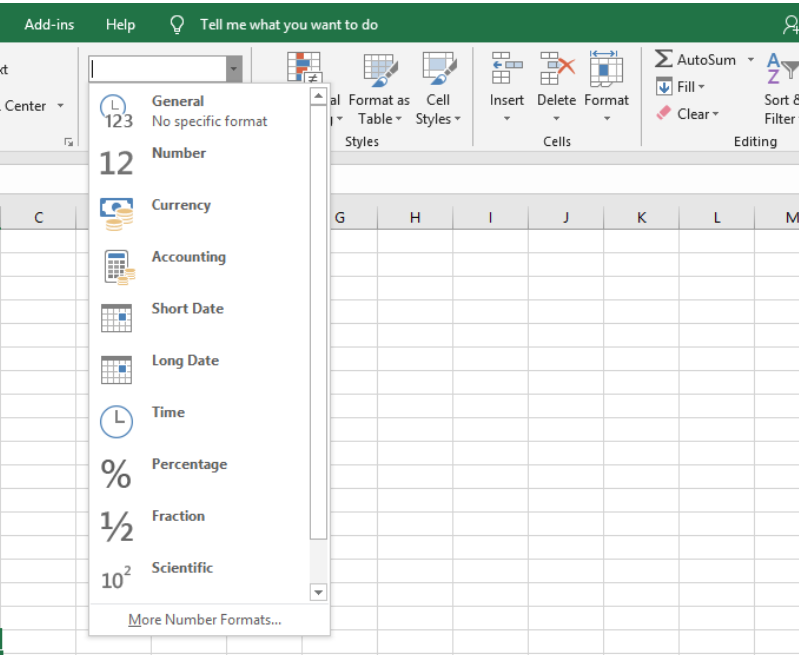Figure 1 – Test questions and answers

### What is Conditional Formatting?

Conditional formatting enables formats within a cell based on the value in it. For instance, if we wish to highlight cells with values less than 40 using the yellow color, then we can use Conditional formatting.

### How to highlight cells with negative values

We can highlight cells with negative values using conditional formatting. We will go to the Home Tab and select the Conditional formatting option. Next, we will go to Highlight Cell Rules and click the “Less than” option. In the Less than Dialog box, we will specify the value we want.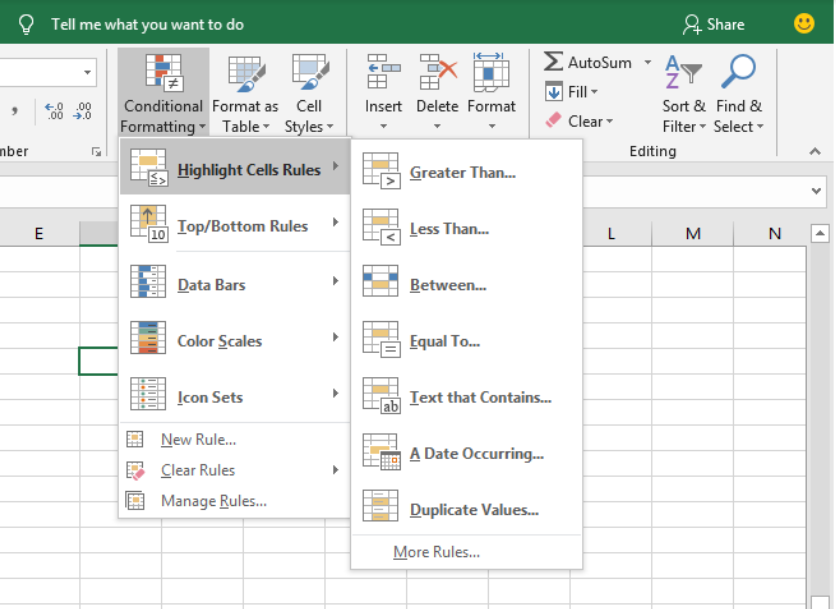Figure 2 – MS excel interview questions and answers

### What is the difference between absolute and relative cell references? In which situations would you use each?

When we have cell references that change as they are copied and pasted around the sheet, we call them, relative cell references. On the other hand, if we have a reference kept static despite being copied around the worksheet, we may call this an absolute reference.  Here are some formats we may see which indicates where we have an absolute reference or relative cell reference.

## Interview Questions on Excel Formula

### What is the order of operations used when evaluating formulas in Excel?

Like Mathematics, Excel uses PEDMAS in carrying out operations. It stands for:

Parentheses

Exponents

Multiplication

Division

Subtraction

### What is the difference between an Excel function and Excel formula?

A function is a built-in operation and involves a specified number of arguments whereas a user creates a formula. A formula may also have multiple functions in it. For example: =A1+A2 is a formula while =SUM(A1:A10) is a function.

### What are the top five functions in Excel? Alternatively, what are the most useful functions in Excel?

Excel has well over 450+ functions and there is no criterion for assigning importance to functions. Every function is important and depends on why the user requires them. However, some of the most commonly used Excel functions include:

• VLOOKUP
• COUNTIF
• SUMIF
• IFERROR
• INDEX / MATCH
• IF Statements
• Pivot Tables

### What are the different kinds of errors seen in Excel?

1. #N/A Error: This is known as the ‘Value Not Available’ error. It occurs when we use a lookup formula and it cannot find the value (or result).
2. #DIV/0! Error: It is called the Division Error. It occurs when Zero (0) divides the figure or value.
3. #VALUE! Error: It is called the Value error. It occurs when there is an incorrect data type in a formula.
4. #REF! Error: This is called the reference error. It occurs when the reference in a formula is no longer valid, for instance, when we have deleted a row/column from our worksheet, which was referred in the formula.
5. #NAME ERROR: This error occurs when we misspell a function.
6. #NUM ERROR: This is called the Number error. It occurs when we calculate a very large value in Excel. For example, =194^643 will return a number error.

### How can we deal with these errors?

1. We can highlight the errors by using the ISERROR function in conditional formatting
2. We can use the IFERROR function to find the specific value in case the formula returns an error.
3. The IFNA function can be used to tackle the #N/A error
4. We can use the ISERROR function to find a FALSE in case there is no error or to return with a TRUE if there is an error in our formula.

## VLOOKUP Questions for Interview

### What is the syntax of the VLOOKUP function?

The syntax of the VLOOKUP function is:

`=VLOOKUP(lookup_value, table_array, col_index_num, range_lookup)`

Where:

• lookup_value defines a value to look up within an external table of data. It can also be a cell reference or text string.
• table_array defines a table of data where we will look up the lookup_value. It could also be named range or range of cells. The lookup_value must appear within the left-most column of the table.
• col_index_num returns with how many columns inside the table_array to look for a value to return.
• range_lookup is a TRUE/FALSE operation that helps specify whether to look for an approximate match or exact match. If omitted, it returns with TRUE for an approximate match.

### Are there any disadvantages of using the VLOOKUP function?

1. Because the col_index_num is inserted manually into the function, VLOOKUP can break if columns of data are entered into the table_array after the function is written. To fix such errors, the VLOOKUP formula may be replaced with the INDEX MATCH, which gives similar functions without this limitation.
2. When VLOOKUP is used on large data sets, it can be very slow.
3. We cannot use the VLOOKUP function if the lookup value is on the right of the value to be returned.
4. VLOOKUP will return with a wrong result if we add or delete a new column in our data.

### What are volatile functions? Give a few examples

Volatile functions recalculate the formula repeatedly whenever there is a change in our worksheet. Some examples of these kinds of functions include:

• Highly volatile: NOW(), RAND(),TODAY()
• Slightly volatile: INDIRECT(), CELL(), OFFSET(),INFO()

### MS. Excel Interview Questions for Excel Keyboard Shortcuts

1. Ctrl + D  – fill contents from one cell to the cells below
2. SHIFT + Spacebar – select an entire row
3. Ctrl + Spacebar – select an entire column
4. Ctrl – open the delete dialog box so we can choose whether to delete cells, rows, or columns
5. Ctrl ;  – inserts a current date
6. Ctrl + Shift ;  – inserts a current time
7. F7 – to spell-check
8. Control F – select the Find Tab from the Find and Replace Dialog box.
9. Control H – select the ReplaceTab from the Find and Replace Dialog box.
10. ALT – To auto sum numbers in a column or row
11. ALT + Enter – insert a new line in the same cell
12. SHIFT + F2 – insert a comment in Excel
13. CTRL + A + A – select the entire worksheet
14. CTRL + N – insert a new Excel workbook

## Excel Pivot Table Interview Questions

### What is a Pivot Table and when should we use one?

We use a Pivot Table to summarize huge data sets easily and it usually involves dragging and dropping rows and column headers to generate reports.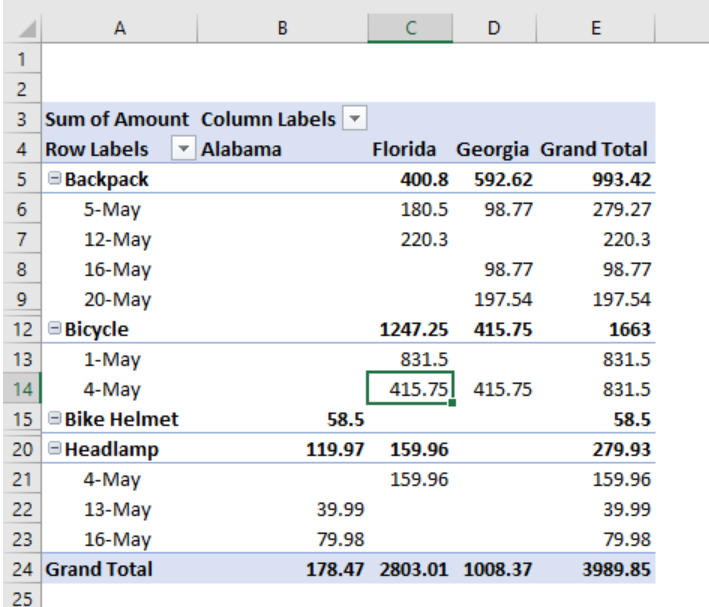Figure 3 – Pivot table

### What are the sections of the Pivot Table?

A Pivot Table has four sections:

1. Value area: This is the area where values are reported
2. Row area: found to the left of the Value area and is made up of the Row headings
3. Columns area: These headings are found at the top of the Value area
4. Filter Area: These are the optional arrows we see which can be used to further screen out some data.

• What is a Pivot Slicer?

A Pivot Table Sliver allows us to filter data when we select one or more options in the slicer box. This feature is found in Excel 2010 and newer.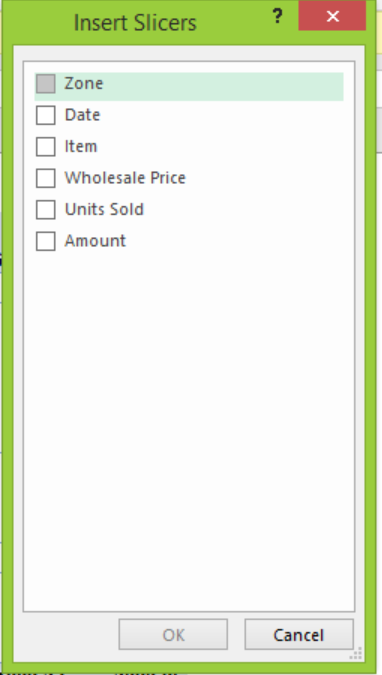Figure 4 – Interview questions on excel

### What are the differences between Regular Charts and Pivot Charts?

Regular charts allow us to do many customizations. Nevertheless, we can only run some limited customizations with Pivot Charts. In addition, if we customize the Pivot Chart, and then update the Pivot Table, we may lose the customization.

### What is a Pivot Cache? Why is it important?

The Pivot Cache is automatically generated when we create the Pivot Table. It holds a replica of our data source, although we cannot see it. It is part of the Pivot Table and connected to it. Whenever we make changes to the Pivot Table, these changes will not alter our data source because these changes will be made in the unseen Pivot Cache. The reason why the Pivot Cache is created is to make our pivot table super-fast when summarizing data even if we have thousands of rows of data.

### What are the calculated fields in Pivot Table?

We use the calculated field to add columns to our Pivot table where we can use existing columns to run calculations.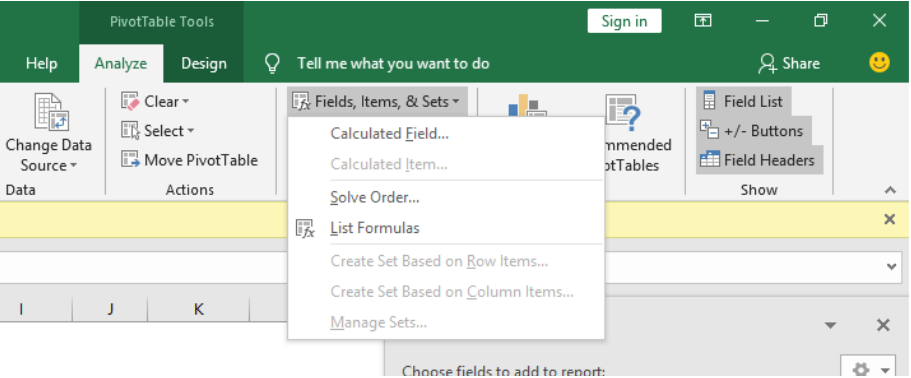Figure 5 – Things to know about excel for an interview

### What are some best practices when creating complex models in Excel?

Excel uses many simple calculations but is most effective when constructing complex mathematical models to help track data over time, project financial results and predict outcomes. However, the best modeling practices is to keep spreadsheets flexible, organized and clean.

## Instant Connection to an Excel Expert

Most of the time, the problem you will need to solve will be more complex than a simple application of a formula or function. If you want to save hours of research and frustration, try our live Excelchat service! Our Excel Experts are available 24/7 to answer any Excel question you may have. We guarantee a connection within 30 seconds and a customized solution within 20 minutes.